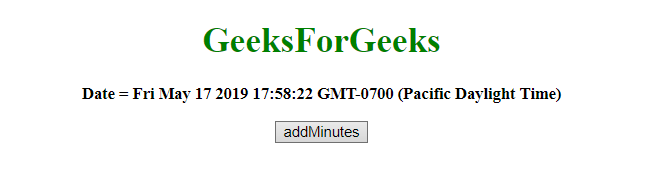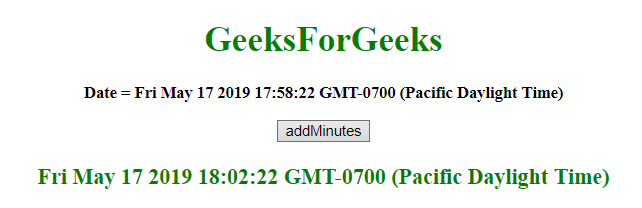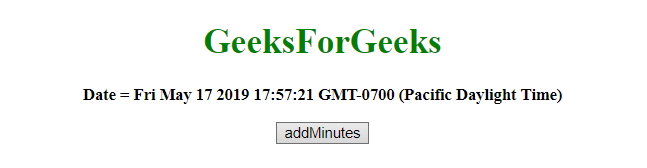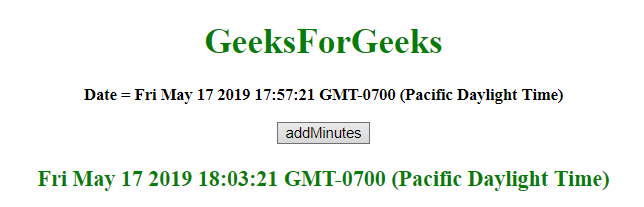# JavaScript | Adding minutes to Date object

Given a date and the task is to add minutes to it. To add minutes to the date object in javascript, some methods are used which are listed below:

• JavaScript getMinutes() Method: This method returns the Minutes (from 0 to 59) of the provided date and time.

Syntax:

```Date.getMinutes()
```

Return value: It returns a number, from 0 to 59, representing the minutes.

• JavaScript setMinutes() Method: This method set the minutes of a date object. This method can also be used to set the seconds and milliseconds.

Syntax:

```Date.setMinutes(min, sec, millisec)
```

Parameters:

• min: It is required parameter. It specifies the integer representing the minutes. Values expected are 0-59, but other values are allowed.
• sec: It is optional parameter. It specifies the integer representing the seconds. Values expected are 0-59, but other values are allowed.
• millisec: It is optional parameter. It specifies the integer representing the milliseconds. Values expected are 0-999, but other values are allowed.

Note: All the previous three parameters accept values apart from their range and these values adjust like:

• min = -1, it means the last minute of the previous hour and same for the other parameters.
• If min value passed to 60, it means the first minute of the next hour and same for the other parameters.

Return value: It returns a number, denoting the number of milliseconds between the date object and midnight January 1 1970.

• JavaScript getTime() method: This method returns the number of milliseconds between midnight of January 1, 1970, and the specified date.

Syntax:

```Date.getTime()
```

Return value: It returns a number, representing the number of milliseconds since midnight January 1, 1970.

• JavaScript setTime() method: This method set the date and time by adding/subtracting a defined number of milliseconds to/from midnight January 1, 1970.

Syntax:

```Date.setTime(millisec)
```

Parameters: It contains single parameter millisec which is required. It specifies the number of milliseconds to be added/subtracted, midnight January 1, 1970.

Return value: It returns, representing the number of milliseconds between the date object and midnight January 1 1970.

Example 1: This example added 4 minutes to the var today by using setTime() and getTime() method.

 `   ` `<``html``>   ` `    ``<``head``>  ` `        ``<``title``>  ` `            ``JavaScript | Adding Minutes to  Date object. ` `        `` ` `    ``  ` `     `  `    ``<``body` `style` `= ``"text-align:center;"` `id` `= ``"body"``>   ` `         `  `        ``<``h1` `style` `= ``"color:green;"` `>   ` `            ``GeeksForGeeks   ` `        `` ` `         `  `        ``<``p` `id` `= ``"GFG_UP"` `style` `= ``"font-size:15px; font-weight:bold;"``> ` `        `` ` `         `  `        ``<``button` `onclick` `= ``"gfg_Run()"``>  ` `            ``addMinutes ` `        `` ` `         `  `        ``<``p` `id` `= ``"GFG_DOWN"` `style` `=  ` `            ``"color:green; font-size: 20px; font-weight: bold;"``> ` `        `` ` `         `  `        ``<``script``> ` `            ``var el_up = document.getElementById("GFG_UP"); ` `            ``var el_down = document.getElementById("GFG_DOWN"); ` `            ``var today = new Date(); ` `            ``el_up.innerHTML = "Date = " + today; ` `             `  `            ``Date.prototype.addMins = function(m) {     ` `               ``this.setTime(this.getTime() + (m*60*1000));  ` `               ``return this;    ` `            ``} ` `             `  `            ``function gfg_Run() { ` `                ``var a = new Date(); ` `                ``a.addMins(4); ` `                ``el_down.innerHTML = a; ` `            ``}          ` `        ``  ` `    ``   ` ` `

Output:

• Before clicking on the button:• After clicking on the button:Example 2: This example adds 6 minutes to the var today by using setMinutes() and getMinutes() method.

 `  ` `<``html``>  ` `    ``<``head``>  ` `        ``<``title``>  ` `            ``JavaScript | Adding Minutes to Date object. ` `        `` ` `    ``  ` ` `  `    ``<``body` `style` `= ``"text-align:center;"` `id` `= ``"body"``>  ` ` `  `        ``<``h1` `style` `= ``"color:green;"` `>  ` `            ``GeeksForGeeks  ` `        `` ` ` `  `        ``<``p` `id` `= ``"GFG_UP"` `style` `= ``"font-size: 15px; font-weight: bold;"``> ` `        `` ` ` `  `        ``<``button` `onclick` `= ``"gfg_Run()"``>  ` `            ``addMinutes ` `        `` ` ` `  `        ``<``p` `id` `= ``"GFG_DOWN"` `style` `= ` `            ``"color:green; font-size: 20px; font-weight: bold;"``> ` `        `` ` `         `  `        ``<``script``> ` `            ``var el_up = document.getElementById("GFG_UP"); ` `            ``var el_down = document.getElementById("GFG_DOWN"); ` `            ``var today = new Date(); ` `            ``el_up.innerHTML = "Date = " + today; ` `             `  `            ``Date.prototype.addMinutes= function(m) { ` `                ``this.setMinutes(this.getMinutes() + m); ` `                ``return this; ` `            ``} ` `             `  `            ``function gfg_Run() { ` `                ``var a = new Date(); ` `                ``a.addMinutes(6); ` `                ``el_down.innerHTML = a; ` `            ``} ` `        ``  ` `    ``  ` `                     `

Output:

• Before clicking on the button:• After clicking on the button:My Personal Notes arrow_drop_upCheck out this Author's contributed articles.

If you like GeeksforGeeks and would like to contribute, you can also write an article using contribute.geeksforgeeks.org or mail your article to contribute@geeksforgeeks.org. See your article appearing on the GeeksforGeeks main page and help other Geeks.

Please Improve this article if you find anything incorrect by clicking on the "Improve Article" button below.